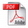# Journal ofFaculty of Civil Engineering

FACULTY JOURNALS
SCIENTIFIC MEETINGS
EBOOKS
ARCHIVE

CONFERENCE PROCEEDINGS
4th INTERNATIONAL CONFERENCE
CONTEMPORARY ACHIEVEMENTS IN CIVIL ENGINEERING 2016 , 2016.y., pp. 709-720

THE LATTICE-BOLTZMANN METHOD IN COMPUTATIONAL FLUID MECHANICSDOI: 10.14415/konferencijaGFS 2016.072 UDC: 532:519.6 CC-BY-SA 4.0 license Author : Horvat, Mirjana; Horvat, Zoltan; Rosić, Nikola; Zindović, Budo Summary: The lattice-Boltzmann method (LBM) is a new method in computational fluid mechanics. While traditional numerical methods directly discretize and solve the macroscopic equations of fluid mechanics, the LBM solves а discrete kinetic equation that reproduces the equations of fluid mechanics in the macroscopic sense. This paper presents in some detail the concept of the distribution function which is essential for the LBM, the Boltzmann equation and the Chapman-Enskog expansion used to reproduce the macroscopic equations of fluid mechanics from the Boltzmann equation. The paper also presents the procedure used for discretizing the Bolzmann equation in velocity and physical space in order to obtain the lattice-Boltzmann equation. Keywords: lattice-Boltzmann method, Chapman-Enskog expansion, computational fluid mechanics